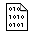## Firefly and PC GAMESS-related discussion club

Learn how to ask questions correctlyRe: E(MP4) - E(MP3) = -26 hartrees?

Alex Granovsky
gran@classic.chem.msu.su

Dear David,
Dear Pedro,

Search this site on "mp4(d)" returns these two useful threads:

http://classic.chem.msu.su/cgi-bin/ceilidh.exe/gran/gamess/forum/?C393fb1c3f2vS-7378-1007-00.htm

http://classic.chem.msu.su/cgi-bin/ceilidh.exe/gran/gamess/forum/?C3d2d5e75bcsK-8577-846+00.htm

In brief, this job has almost linearly dependent basis set. As printed
in the output file:

``` THE OVERLAP MATRIX HAS    4 EIGENVALUES BELOW 1.1E-04
THE SMALLEST OF THESE IS 1.35832E-09.
```

The eigenvalue of overlap matrix of 1.35832E-09 corresponds
to a severe quasi-linear dependence.

The sensitivity of MP4(D) term of the MP4 energy to the linear
dependence in the AO basis set has been already discussed on this
forum several times. The MP4(D) term is the most sensitive one
because during its computation one needs to transform integrals and
other quantities from AO to MO basis, then back from MO to AO,
and finally from AO to MO again. Each transformation kills up to
several significant digits of the result (more precisely,
the number of digits lost is directly related to the condition
number of the overlap matrix) if all orbitals (including virtual
ones) are not computed very precisely. So in the worst case like
this one, one can lost all significant digits.

What to do?

One need to delete some orbitals to increase the smallest
eigenvalue of the overlap matrix.

As output indicates, the most problematic orbital is:

```   0.2857E-08   -    C   5  S,   SHELL  31,   AO   73
```

i.e. the S part of the diffuse L shell on the atom C5

I attached archive with modified input file as well as
the corresponding output file. In the new input, the
problematic orbital was removed by replacing L shell
located on atom C5 by P shell. Otherwise, the \$DATA
group of the modified input is equivalent to the initial
\$DATA and \$BASIS groups.

I also increased ICUT and ITOL, and disabled fdiff.
Finally, I tightened nconv to 6 as recommended in
output.

The smallest eigenvalue of overlap matrix is now:

``` THE SMALLEST OF THESE IS 4.10644E-08.
```

i.e. ca. 30 times better!

As a result, we obtain:

``` RESULTS OF MOLLER-PLESSET 4TH ORDER CORRECTION ARE

E(RHF)       =      -191.5685568750

E(D,2)       =        -0.6457464825
E(MP2)       =      -192.2143033575

E(D,3)       =        -0.0166674371
E(D,2+3)     =        -0.6624139197
E(MP3)       =      -192.2309707947

E(S,4)       =        -0.0073886039
E(D,4)       =        -0.0200238070
E(D,2+3+4)   =        -0.6824377267
E(T,4)       =        -0.0337953970
E(R+Q,4)     =         0.0168396781
E(SDTQ,4)    =        -0.0443681298
E(SDTQ,2+3+4)=        -0.7067820495
E(MP4-SDTQ)  =      -192.2753389245

```

Hope this helps.

Kind regards,
Alex Granovsky

On Mon Apr 4 '16 0:12am, David Shobe wrote
------------------------------------------
>Is this the correct MP4 energy for this molecule (1,3-pentadiyne)?

> RESULTS OF MOLLER-PLESSET 4TH ORDER CORRECTION ARE

>            E(RHF)       =      -191.5694925024

>            E(D,2)       =        -0.6494633272
>            E(MP2)       =      -192.2189558295

>            E(D,3)       =         0.2696414733
>            E(D,2+3)     =        -0.3798218539
>            E(MP3)       =      -191.9493143563

>            E(S,4)       =        -0.0517477560
>            E(D,4)       =       -26.2790188653
>            E(D,2+3+4)   =       -26.6588407192
>            E(T,4)       =        -0.0344433158
>            E(R+Q,4)     =         0.0171693598
>            E(SDTQ,4)    =       -26.3480405772
>            E(SDTQ,2+3+4)=       -26.7278624311
>            E(MP4-SDTQ)  =      -218.2973549335
>This message contains the 56 kb attachment [ input_with_fixed_mp4_problem.zip ]Wed Apr 6 '16 10:07pmThis message read 476 times